# Precalculus : Find the Eccentricity of a Hyperbola

## Example Questions

← Previous 1 2 Next →

### Example Question #11 : Find The Eccentricity Of A Hyperbola

Find the eccentricity for the following hyperbola: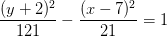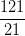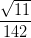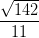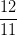Explanation:

Recall the standard form of the equation of a vertical hyperbola: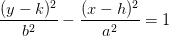, where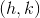is the center

For a horizontal hyperbola, use the following equation to find the eccentricity: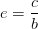, where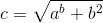For the given hyperbola,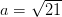and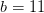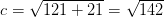Thus,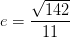### Example Question #31 : Hyperbolas

Find the eccentricity for the following hyperbola: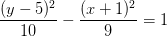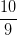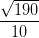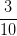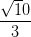Explanation:

Recall the standard form of the equation of a vertical hyperbola:, whereis the center

For a horizontal hyperbola, use the following equation to find the eccentricity:, whereFor the given hyperbola,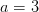and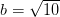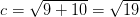Thus,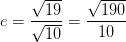### Example Question #13 : Find The Eccentricity Of A Hyperbola

Find the eccentricity of the following hyperbola: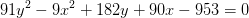Explanation:

Recall the standard form of the equation of a vertical hyperbola:, whereis the center

We need to put the given equation into the standard form of the equation.

Start by grouping like terms together.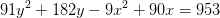Factor out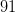from the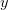terms and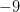from theterms.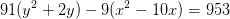Complete the squares. Remember to add the same amount to both sides of the equation!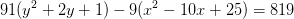Divide both sides by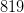.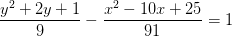Factor both terms to get the standard equation.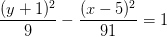For a horizontal hyperbola, use the following equation to find the eccentricity:, whereFor the given hyperbola,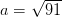and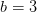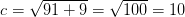Thus,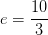← Previous 1 2 Next →

### All Precalculus Resources